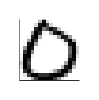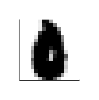Julia & graphic enthusiast. Interested in high performance computing, GPUs and machine learning.

# Homework 4

The data we will use is a simplified and reduced version of the MNIST set of handwritten digits, one of the most commonly-used data sets in machine learning.

This version of the data was kindly provided by Prof. Raj Rao, University of Michigan.

(i) Load the training data from using the JLD.jl package, and explore it.

The data consists of a 3-dimensional array ("tensor"):

• The third component runs from 1 to 10, and specifies the digit from 0 to 9.
• The columns $\mathbf{x}_i$ (the first component) are vectors of length $n=256$ representing grayscale images of $16 \times 16$ pixels.
• The second component runs over the number of training examples.

In the same file are the corresponding labels $y_i \in {0, \ldots, 9} =: Y$.

Load the test data from .

training_digits.jld
test_digits.jld
import JLD
# reference an uploaded file via the @ context menu
test = JLD.load(test_digits.jld↩);

(ii) Visualize some of the digits by reshaping them to a $16 \times 16$ array and plotting the resulting image, e.g. using matshow in PyPlot. Use e.g. @manipulate. Note that some of the data is all zero.

using Interact, WebSockets, WebIO
using Plots
gr(leg=false, ticks=nothing, grid=false, border=false);
@manipulate for digit=0:9, idx=1:319
heatmap(rotl90(reshape(train[:,idx,digit+1], 16, 16)),
aspectratio=:equal, size=(100, 100))
end
good_idxs = Int[]
raw_digits = Int[]
for i in 1:3190
digit = Int(floor((i-1)/319))
idx = i - 319*digit
if !all(train[:,idx,digit+1] .== 0)
append!(good_idxs, i)
append!(raw_digits, digit)
end
end

digits = [d == digit+1 ? 1 : 0 for digit in raw_digits, d in 1:10];
anim = @animate for i in good_idxs
digit = Int(floor((i-1)/319))
idx = i - 319*digit
heatmap(rotl90(reshape(train[:,idx,digit+1], 16, 16)),
aspectratio=:equal, size=(100, 100))
end every 10
gif(anim, "/results/res.gif", show_msg  = false);## 2. Least squares

One of the first ideas that we could have is to look for a simple function $f^{(i)}$ that classifies each digit as e.g. "being a 7 or not being a 7". We could thus look for a linear map (the simplest type of map) from $\mathbb{R}^n$ to $\mathbb{R}$ that maps $\mathbf{x}_i$ to a $1$ or $0$, depending if the image does or does not correspond to a digit of type $i$.

(iii) Express the "being a 7" problem as a matrix-vector multiplication over a single matrix containing all the data. Use least squares (\\) to solve the problem.

begin Ned

size(W) = 10, 256

size(x) = 256, 1707

size(y) = 10, 1707

end Ned

A = zeros(1707, 256)

for i in 1:length(good_idxs)
gidx = good_idxs[i]
digit = Int(floor((gidx-1)/319))
idx = gidx - 319*digit
A[i,:] = train[:,idx,digit+1]
end
x = A'
y = digits'
W = y / x;

W' ≈ x' \ y'  # using backslash notation

(iv) Stacking up all such problems vertically gives a problem where the vector $\mathbf{x}_i$ is mapped onto a one-hot vector $\mathbf{y}_i$ representing the digit $y_i$. It turns out that some of the data vectors are all zero. Remove these and make one single matrix $\mathsf{x}$ by horizontally stacking the data, and another, $\mathsf{y}$ the corresponding one-hot vectors.

Using least squares, find the matrix $\mathsf{W}$ that best solves $\mathsf{W} \mathbf{x}_i \approx \mathbf{y}_i$ for each $i$. (NB: Take care about the dimensions. What are you solving for?)

Use the resulting matrix $\mathsf{W}$ to classify the test digits. What proportion does it get right?

using Printf
y_raw = W*x;
y_hat = mapslices(argmax, y_raw, dims = 1) .- 1

incorrect_idxs = findall(x -> x != 0, (y_hat - raw_digits'))
@printf("Got %.1f%% right in training set", 100*(1-length(incorrect_idxs)/length(y_hat)))
y_raw = W*test["digits"];
y_hat = mapslices(argmax, y_raw, dims = 1) .- 1

incorrect_idxs = findall(x -> x != 0, vec(y_hat - test["labels"]))
@printf("Got %.1f%% right in testing set", 100*(1-length(incorrect_idxs)/length(y_hat)))
sorted_incorrect_idxs = sort(incorrect_idxs, by= i->test["labels"][i])
anim = @animate for idx in sorted_incorrect_idxs
heatmap(rotl90(reshape(test["digits"][:,idx], 16, 16)),
aspectratio=:equal, size=(100, 100))
end
gif(anim, "/results/res.gif", show_msg  = false);(v) Instead of just $\mathsf{W} \mathbf{x}_i = \mathbf{y}_i$, do the same with $\mathsf{W} \mathbf{x} + \mathbf{b} = \mathbf{y}_i$, by adding an extra $1$ at the bottom of each $\mathbf{x}_i$.

x_b = [x; ones(1, size(x, 2))]
W_b = y / x_b

y_raw = W_b*[test["digits"]; ones(1, size(test["digits"], 2))]
y_hat = mapslices(argmax, y_raw, dims = 1) .- 1

incorrect_idxs = findall(x -> x != 0, vec(y_hat - test["labels"]))
@printf("Got %.1f%% right in testing set", 100*(1-length(incorrect_idxs)/length(y_hat)))

begin Ned

Why does this make no difference? Perhaps because the corners tended to be -1.0 already, and so were useful as constant factors?

end Ned

(vi) Use @manipulate to scroll through misclassified images and discuss their features.

incorrect_by_label = [filter(i -> test["labels"][i] == j, incorrect_idxs) for j in 0:9]
tfont = Plots.Font("Arial", 4, :hcenter, :vcenter, 0.0, RGB(0,0,0))
@manipulate for i in 0:9
plots = [heatmap(rotl90(reshape(test["digits"][:,idx], 16, 16)),
aspectratio=:equal,
title=string(idx), titlefont=tfont)
for idx in incorrect_by_label[i+1]]
n = length(incorrect_by_label[i+1])
plot(plots..., size=(50*sqrt(n), 50*sqrt(n)))
end
test["digits"] # output the array, so that we're able to reference it!

### 2.1. Create a plot with Python using the above Julia Data

digits = nil↩ # reference the above julia array
import matplotlib
import matplotlib.pyplot as plt
import numpy as np
x = np.matrix(digits)
img = np.reshape(x[2, :], (16, 16))
fig, ax = plt.subplots()
plt.imshow(img, cmap = 'Greys')
fig